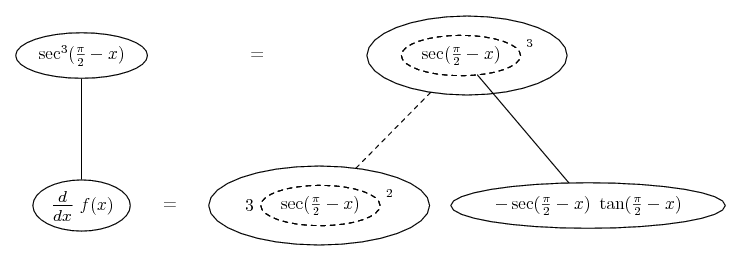# Thread: Double Chain Rule Problem

1. ## Double Chain Rule Problem

Hi everyone. I'm trying to derive this equation, but I keep getting the wrong answer. I would appreciate any help : )

f(x)=
$\displaystyle sec^3(pi/2-x)$

So I apply the chain rule:

f'(x)=
$\displaystyle 3*(sec^2(pi/2-x)) * (sec^2(pi/2-x))' * (pi/2-x)'$

f'(x)=
$\displaystyle -6*(sec^2(pi/2-x) *(tan(pi/2-x)) * (sec^2(pi/2-x))$

However, the math program I use gives me a different answer. Does anyone see where I went wrong?

2.Originally Posted by bobbert116Hi everyone. I'm trying to derive this equation, but I keep getting the wrong answer. I would appreciate any help : )

f(x)=
$\displaystyle sec^3(pi/2-x)$

So I apply the chain rule:

f'(x)=
$\displaystyle 3*(sec^2(pi/2-x)) * (sec^2(pi/2-x))' * (pi/2-x)'$

f'(x)=
$\displaystyle 3*(sec^2(pi/2-x) * 2*(tan(pi/2-x)) * (sec^2(pi/2-x)) * -1$

However, the math program I use gives me a different answer. Does anyone see where I went wrong?
I've highlighted the problem in the middle row. Can't see how you got from there to the next, either. Anyway, sec not sec^2 is the next function in from the outside of your first function.

Edit:

Just in case a picture helps...... where... is the chain rule. Straight continuous lines differentiate downwards (integrate up) with respect to x, and the straight dashed line similarly but with respect to the dashed balloon expression (the inner function of the composite which is subject to the chain rule).

_________________________________________

Don't integrate - balloontegrate!

Balloon Calculus; standard integrals, derivatives and methods

Balloon Calculus Drawing with LaTeX and Asymptote!

3. Hello, bobbert116!

It's your derivative of secant . . .

Differentiate: .$\displaystyle f(x)\:=\:\sec^3\left(\tfrac{\pi}{2}-x\right)$

Derivative of $\displaystyle \sec^3\left(\tfrac{\pi}{2}-x\right) \quad\Rightarrow\quad 3\sec^2\!\left(\tfrac{\pi}{2}-x\right)$

Derivative of $\displaystyle \sec\left(\tfrac{\pi}{2}\right) \quad\Rightarrow\quad {\color{blue}\sec\left(\tfrac{\pi}{2}-x\right)\tan\left(\tfrac{\pi}{2}-x\right)} \;\;{\color{red}\Leftarrow\text{ Here!}}$

Derivative of $\displaystyle \left(\tfrac{\pi}{2}\right) \quad\Rightarrow\quad -1$

,

,

,

# double chain formula

Click on a term to search for related topics.

#### Search Tags

chain, double, problem, rule